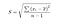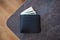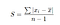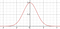# Standard Deviation is an Inferior Measurement*

## *if you don’t need to use calculus…

Standard deviation: the best metric to use if you want to find the average distance from the mean in a dataset, right?

This is wrong on two accounts, actually. Standard deviation is neither the best metric nor is it purely the average distance between values in a dataset. To explore these claims, let’s take a closer look at the formula.sample standard deviation (ignore the -1 in the denominator for now)

This looks tricky, but we can break it down.

1. Subtract the mean from the data point.
2. Take the resulting difference and square it.
3. Repeat steps 1–2 for the rest of the data in the sample and add all the products of step 2 together.
4. Divide by the number of data points in the sample (let’s ignore the -1 for now, it isn’t relevant to this discussion).
5. Take the square root.

In a normal average, you do not square your values (step 2) and then take the square root after you finish dividing (step 5), so what gives?

To answer this, imagine that we measure the amount of money in a random sample of three peoples’ wallets (this sample size is too small to draw meaningful conclusions in real statistical analysis, but bear with me for the purpose of the thought experiment). Person one has \$5, person two has \$10, and person three has \$15. Let’s try taking the standard deviation without squaring or taking the square root.Photo by Emil Kalibradov on Unsplash
1. \$5-\$10 (the mean) is \$-5.
2. \$10–\$10 (the mean) is \$0.
3. \$15-\$10 (the mean) is \$5.
4. We add 1 through 3 up (0) and divide by our sample size (3) to get a standard deviation of … \$0?

Ok, fine. The differences from the mean all have to share the same sign (+ or -) before we add if we want to get a real answer, so the rationale is that we square the numbers to fix the signs and then take the square root at the end to bring our answer back down to normal size, but here’s the problem:

Allow me to illustrate. Let’s say that we measure the money in the wallets of 30 new people. Most of them have \$2. 2² = 4. That’s two times as big as two. But one person in our sample has \$100 in their wallet. 100² = 10,000. That’s 100 times as big as 100.

There’s no doubt the person with the \$100 bill will skew the mean, but to the extent that standard deviation squares numbers, the \$100 will skew the standard deviation even more.

So standard deviation is actually a weighted average that emphasizes extreme data points (which you don’t want if the goal is to accurately describe most of the data). Why can’t we just have a normal average that places equal weight on every data point?

Why don’t we just take the absolute value of the difference between the data point and the mean and forego the business with exponents?mean absolute deviation: the formula that results when you take the absolute value and forego the business with exponents

Well, the convention has stuck, thanks to Sir Ronald Fisher. In the early 1900s, he wrote an influential paper that claimed that given a random sample of a population with a perfectly normal distribution, standard deviation is a more accurate measure of population deviation than absolute mean deviation¹.A perfectly normal distribution: very unlikely in practice

In the real world, unfortunately, perfectly normal distributions are exceedingly improbable. As population size increases, the probability of that population being entirely symmetrical and unimodal (read: perfectly normal) gets closer to zero.

Given a real sample, absolute mean deviation more accurately measures the population deviation than standard deviation, not the other way around.

## Conclusion: Use mean absolute deviation, not standard deviation. It’s better*.

-

*Absolute values do not play nice with calculus. I won’t delve here, but absolute value functions are not differentiable (solvable) at x = 0.² If you want to construct a regression (create a line of best fit for your data), you need to use standard deviation. The regression formula relies upon your ability to take the derivative at x = 0. Provided you only want to analyze the average variance from the mean (i.e. what standard deviation is advertised to do), you should use absolute mean deviation.

## Math Simplified

Simplified is a publication aiming at making mathematics accessible and enjoyable.## Math Simplified

Simplified is a publication aiming at making mathematics accessible and enjoyable.

## More From Medium

Medium is an open platform where 170 million readers come to find insightful and dynamic thinking. Here, expert and undiscovered voices alike dive into the heart of any topic and bring new ideas to the surface. Learn more

Follow the writers, publications, and topics that matter to you, and you’ll see them on your homepage and in your inbox. Explore

If you have a story to tell, knowledge to share, or a perspective to offer — welcome home. It’s easy and free to post your thinking on any topic. Write on Medium